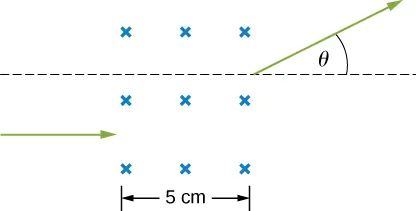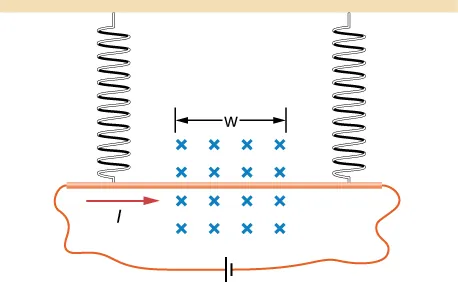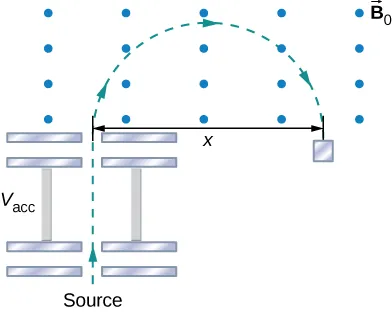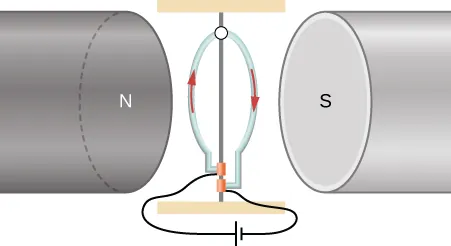University Physics Volume 2

# Challenge Problems

University Physics Volume 2Challenge Problems

### Challenge Problems

102.

A particle of charge +q and mass m moves with velocity $v→0v→0$ pointed in the +y-direction as it crosses the x-axis at x = R at a particular time. There is a negative charge –Q fixed at the origin, and there exists a uniform magnetic field $B→0B→0$ pointed in the +z-direction. It is found that the particle describes a circle of radius R about –Q. Find $B→0B→0$ in terms of the given quantities.

103.

A proton of speed v = $6×105m/s6×105m/s$ enters a region of uniform magnetic field of B = 0.5 T at an angle of $q=30°q=30°$ to the magnetic field. In the region of magnetic field proton describes a helical path with radius R and pitch p (distance between loops). Find R and p.

104.

A particle’s path is bent when it passes through a region of non-zero magnetic field although its speed remains unchanged. This is very useful for “beam steering” in particle accelerators. Consider a proton of speed $4×106m/s4×106m/s$ entering a region of uniform magnetic field 0.2 T over a 5-cm-wide region. Magnetic field is perpendicular to the velocity of the particle. By how much angle will the path of the proton be bent? (Hint: The particle comes out tangent to a circle.)105.

In a region a non-uniform magnetic field exists such that $Bx=0,By=0,andBz=ax,Bx=0,By=0,andBz=ax,$ where a is a constant. At some time t, a wire of length L is carrying a current I is located along the x-axis from origin to x = L. Find the magnetic force on the wire at this instant in time.

106.

A copper rod of mass m and length L is hung from the ceiling using two springs of spring constant k. A uniform magnetic field of magnitude $B0B0$ pointing perpendicular to the rod and spring (coming into the page in the figure) exists in a region of space covering a length w of the copper rod. The ends of the rod are then connected by flexible copper wire across the terminals of a battery of voltage V. Determine the change in the length of the springs when a current I runs through the copper rod in the direction shown in figure. (Ignore any force by the flexible wire.)107.

The accompanied figure shows an arrangement for measuring mass of ions by an instrument called the mass spectrometer. An ion of mass m and charge +q is produced essentially at rest in source S, a chamber in which a gas discharge is taking place. The ion is accelerated by a potential difference $VaccVacc$ and allowed to enter a region of constant magnetic field $B→0.B→0.$ In the uniform magnetic field region, the ion moves in a semicircular path striking a photographic plate at a distance x from the entry point. Derive a formula for mass m in terms of $B0,B0,$ q, $Vacc,Vacc,$ and x.108.

A wire is made into a circular shape of radius R and pivoted along a central support. The two ends of the wire are touching a brush that is connected to a dc power source. The structure is between the poles of a magnet such that we can assume there is a uniform magnetic field on the wire. In terms of a coordinate system with origin at the center of the ring, magnetic field is $Bx=B0,By=Bz=0,Bx=B0,By=Bz=0,$ and the ring rotates about the z-axis. Find the torque on the ring when it is not in the xz-plane.109.

A long-rigid wire lies along the x-axis and carries a current of 2.5 A in the positive x-direction. Around the wire is the magnetic field $B→=2.0i^+5.0x2j^,B→=2.0i^+5.0x2j^,$ with x in meters and B in millitesla. Calculate the magnetic force on the segment of wire between x = 2.0 m and x = 4.0 m.

110.

A circular loop of wire of area 10 cm2 carries a current of 25 A. At a particular instant, the loop lies in the xy-plane and is subjected to a magnetic field $B→=(2.0i^+6.0j^+8.0k^)×10−3T.B→=(2.0i^+6.0j^+8.0k^)×10−3T.$ As viewed from above the xy-plane, the current is circulating clockwise. (a) What is the magnetic dipole moment of the current loop? (b) At this instant, what is the magnetic torque on the loop?

Order a print copy

As an Amazon Associate we earn from qualifying purchases.